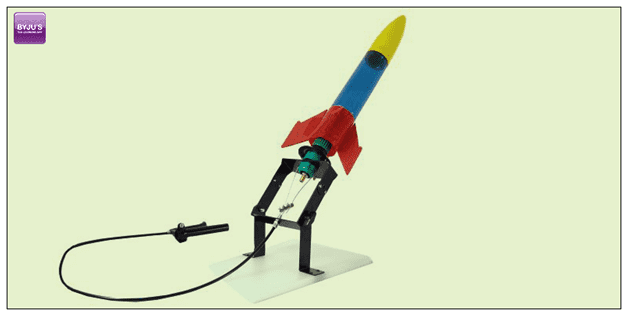# Rocket Science

You might have heard the phrase “It is not rocket science” tossed around some time or the other. When someone says “It’s not rocket science,” what they really mean is that something is not so difficult. Rocket scientists are brilliant people, but rocket science is based on concepts that we understand. The same basic science principle and laws work in both NASA rockets and small paper ones.

## What Is Rocket Science?Rocket science is one of the branches of aerospace engineering. It plays a crucial part in the modern world. From launching satellites to testing ballistic missiles, rocket science has innumerable applications. The history of rockets dates back to the first century when rockets were used by the Chinese to ward off evil spirits. Since then the rocket has evolved tremendously. The propulsion of rockets is based on a fundamental kind of motion and one needs to be familiar with Newton’s Laws of Motion to understand it.

## What Is The Formula For Rocket Science?

The formula used for rocket science is known as the Tsiolkovsky rocket equation or ideal rocket equation. The formula explains the motion of vehicles based on acceleration and using its thrust to get high velocity which is on the basis of conservation of momentum. The equation is given as:

 $\Delta v=v_{e}ln\frac{m_{0}}{m_{f}}=I_{sp}g_{0}ln\frac{m_{0}}{m_{f}}$

Where,

• Δv: maximum change of velocity of the vehicle
• m0: initial total mass which is also known as wet mass (with propellant)
• mf: final total mass which is also known as dry mass (without propellant)
• ve: Ispg0 is the effective exhaust velocity
• Isp: specific impulse in a dimension of time
• g0: standard gravity
• ln: natural logarithm function

## What Are The Fuels Used In Rocket?

The two primary fuels used in the rocket are:

• Liquid: Liquid fuel is most widely used and is composed of fuel and oxidizer. The most commonly used liquid fuel is liquid hydrogen as fuel and liquid oxygen as an oxidizer.
• Solid: Solid rocket fuels are preferred less than they lack control and efficiency. These are also composed of two parts like liquid fuel, but they are in solid-state. Ammonium nitrate is an example of solid fuel.

## How Does Rocket Fuel Burn-In Space Without Oxygen?

As we know that the rocket fuel is composed of fuel and an oxidizer, these two are ignited in the combustion chamber resulting in the expansion of the gases. The pressure in the gas hit harder on the top making the rocket move forward. The exhaust is released out from the bottom and the pressure here is less.

## What Causes Thrust In A Rocket?

The amount of thrust produced by the rocket depends on three parameters:

• The mass flow rate (m dot) of through the engine
• The exit velocity (Ve) of the exhaust
• The pressure at the nozzle exit (pe)

The thrust of the system is explained using “thrust equation”

 F=m dot*Ve+(pe-p0)*Ae

Where,

• F is the thrust
• m dot is the mass flow rate
• Ve is the exit velocity
• pe is the exit pressure
• p0 is the free stream pressure
• Ae is the area ratio of the throat to exit

## Rocket Experiment

### Objective

This experiment is a simple example of an application of pressure where the experiment can be done with the use of simple household things.

### Materials

• Paint or water coloring. It is optional.
• A container of Water.
• Blue- Tak, small piece
• A photo film canister. One can get in an old camera for free.
• A tablet – Alka Seltzer.

### Procedure

• Remove the cap of a canister. Paste the blue-tak inside the camera film canister.
• Now paste the Alka seltzer carefully to the blue-tak.
• Add water to the canister. Note it should be filled just half the canister.
• Close the lid tightly.
• Now you have fully loaded pocket rocket.
• Invert the canister (upside down) and once done step back.

### Result

Once the above procedure is done carbon-dioxide starts releasing as Alka Seltzer dissolves. Gradually there will an increase in the amount of carbon dioxide released. Since the camera film canister is airtight, there isn’t any gap to escape. As a result, the pressure will be created within the canister. Finally, it pops out.